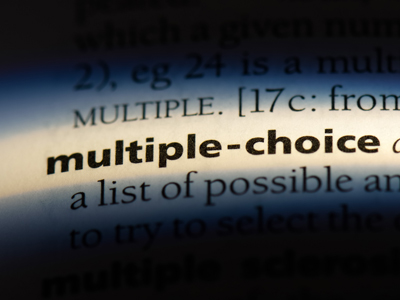This is a multiple-choice quiz, but what is a multiple in maths?

# Pick a Figure 2

Welcome to the second of our Picking a Figure to Fulfil Criteria quizzes. If you have already played the first then you will know what to expect. Basically, you will be asked to spot prime numbers, factors, square numbers and multiples. If you can do that then these kinds of question will be easy when you come to your Eleven Plus Verbal Reasoning exam.

Prime numbers are ones which can only be divided by 1 and by themselves, without leaving a remainder. There is only one even prime number, the number 2. So, if you are looking for a prime number and you see even numbers in the list of options, you can discard these right away. That should speed things up a bit and save valuable time when you come to take your test.

Saving time is a good thing, but not if it costs you. Make sure that you read each question thoroughly and understand exactly what is required before you choose your answers. Good luck!

Example:
Which of the following is not a factor of 24?

2, 6, 4, 48, 12 Answer:
‘Factor’ means a number which fits exactly into another. The numbers 2, 4, 6 and 12 all fit into 24 whereas 48 does not, so the correct answer is 48.
1.
Which of the following is a factor of 62?

31, 4, 21, 14, 32
4
31
14
21
Factors are numbers which divide into another number without leaving a remainder. The factors of 62 are 1, 2, 31 and 62. The only one of these amongst the options is 31
2.
Which of the following is a factor of 67?

3, 13, 1, 33, 21
13
3
21
1
Factors are numbers which divide into another number without leaving a remainder. The factors of 67 are 1 and 67 (which means it is a prime number). None of the other numbers have 67 in their times tables
3.
Which of the following is not a multiple of 8?

32, 72, 36, 16, 48
36
72
16
48
Multiples are numbers you find after multiplying another number. More simply, if a number is in another number’s times table, then it is a multiple of that number. To quickly see if a number is a multiple of another, divide it by the other number and, if there is no remainder, it is a multiple.
All of the options, except 36, can be divided by 8 with no remainder
4.
Which of the following is not a square number?

49, 122, 64, 16, 9
9
64
122
49
Square numbers are what we get when we multiply a number by itself. All of the options are square numbers (3 x 3 = 9, 4 x 4 = 16, 7 x 7 = 49, 8 x 8 = 64) but 122 is not
5.
Which of the following is a square number?

62, 36, 24, 91, 12
32
91
36
24
Square numbers are what we get when we multiply a number by itself. 36 is the square of 6 because 6 x 6 = 36. None of the other options are square numbers
6.
Which of the following is a prime number?

35, 63, 41, 57, 8
63
41
35
8
Prime numbers can only be divided by two numbers (one and themselves) without leaving a remainder. They will only appear in the 1 times table and their own times table, but in no others. The number 41 is the only option which can meet these requirements
7.
Which of the following is not a multiple of 6?

24, 42, 18, 54, 76
76
42
54
18
Multiples are numbers you find after multiplying another number. More simply, if a number is in another number’s times table, then it is a multiple of that number. To quickly see if a number is a multiple of another, divide it by the other number and, if there is no remainder, it is a multiple.
All of the options, except 76, can be divided by 6 with no remainder
8.
Which of the following is not a factor of 52?

4, 13, 14, 26, 52
26
14
4
13
Factors are numbers which divide into another number without leaving a remainder. The factors of 52 are 1, 2, 4, 13, 26 and 52. The number 14 is not a factor of 52, because 52 does not appear in the 14 times table
9.
Which of the following is not a factor of 45?

15, 9, 3, 90, 5
15
9
90
3
Factors are numbers which divide into another number without leaving a remainder. The factors of 45 are 1, 3, 5, 9, 15 and 45. The number 90 is not a factor of 45, but 45 is a factor of 90
10.
Which of the following is a multiple of 12?

34, 84, 26, 18, 52
26
52
34
84
Multiples are numbers you find after multiplying another number. More simply, if a number is in another number’s times table, then it is a multiple of that number. To quickly see if a number is a multiple of another, divide it by the other number and, if there is no remainder, it is a multiple.
The only one of the options which can be divided by 12 with no remainder is 84
Author:  Stephen O'Hara and Ian Miles